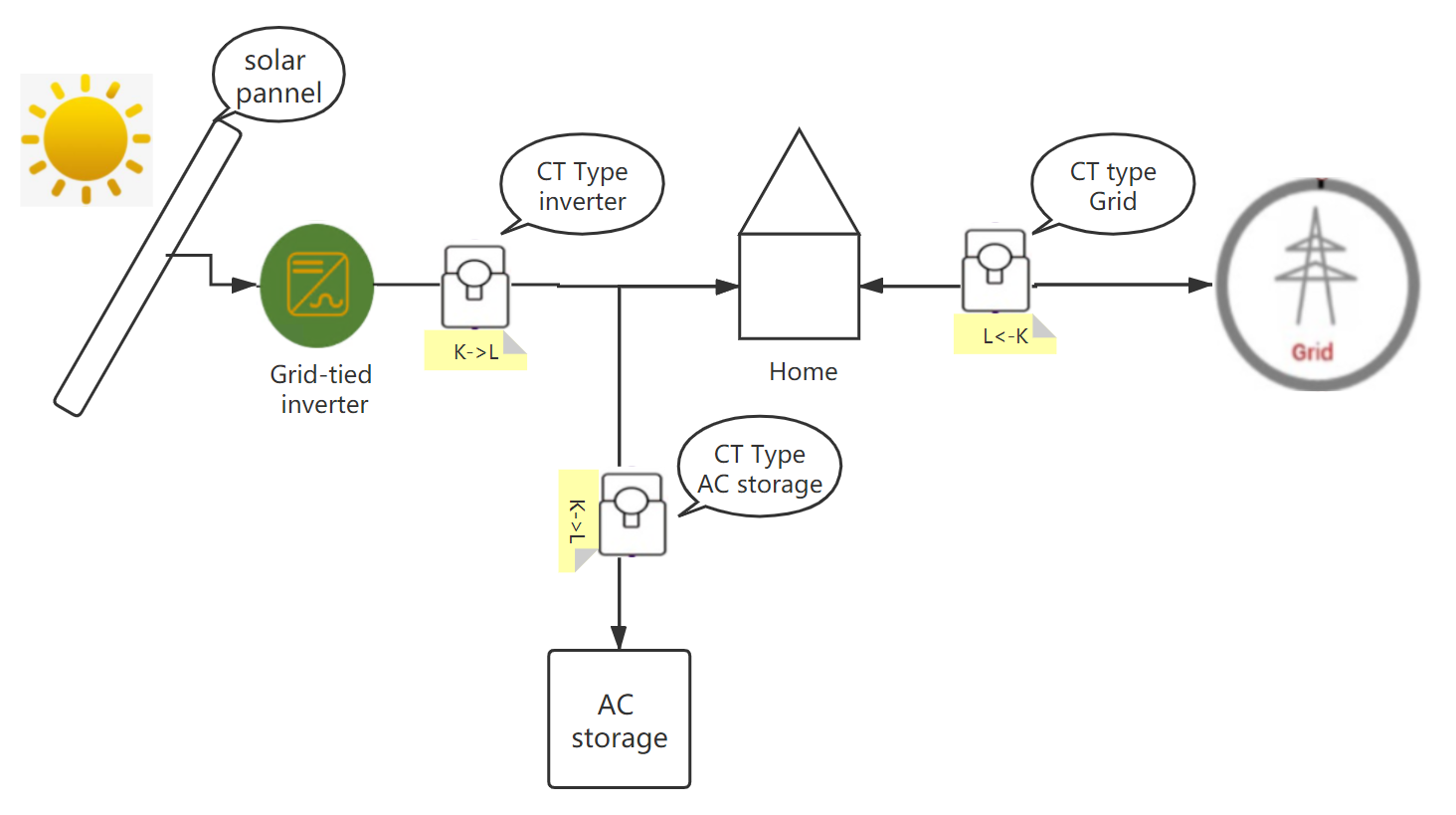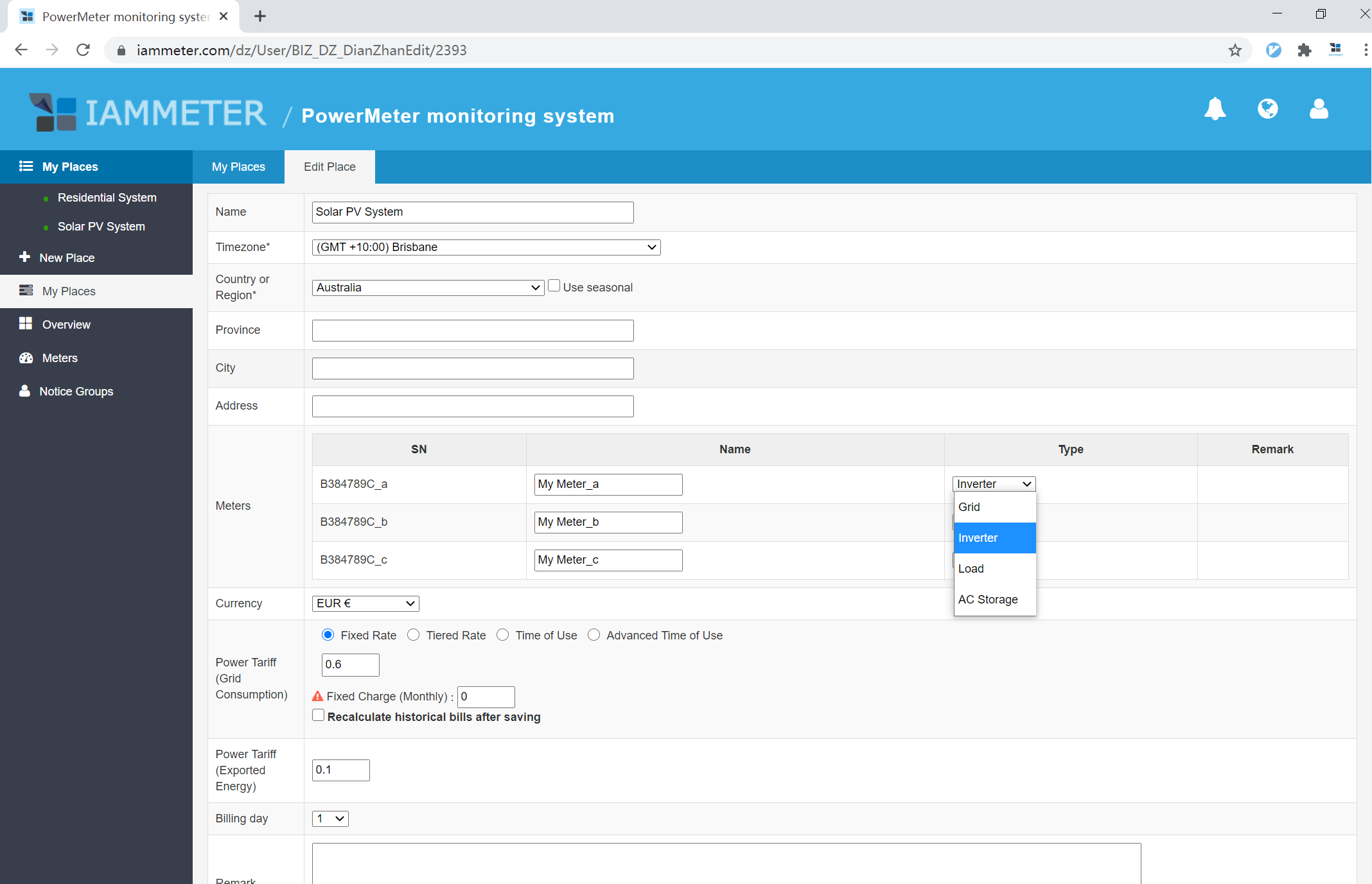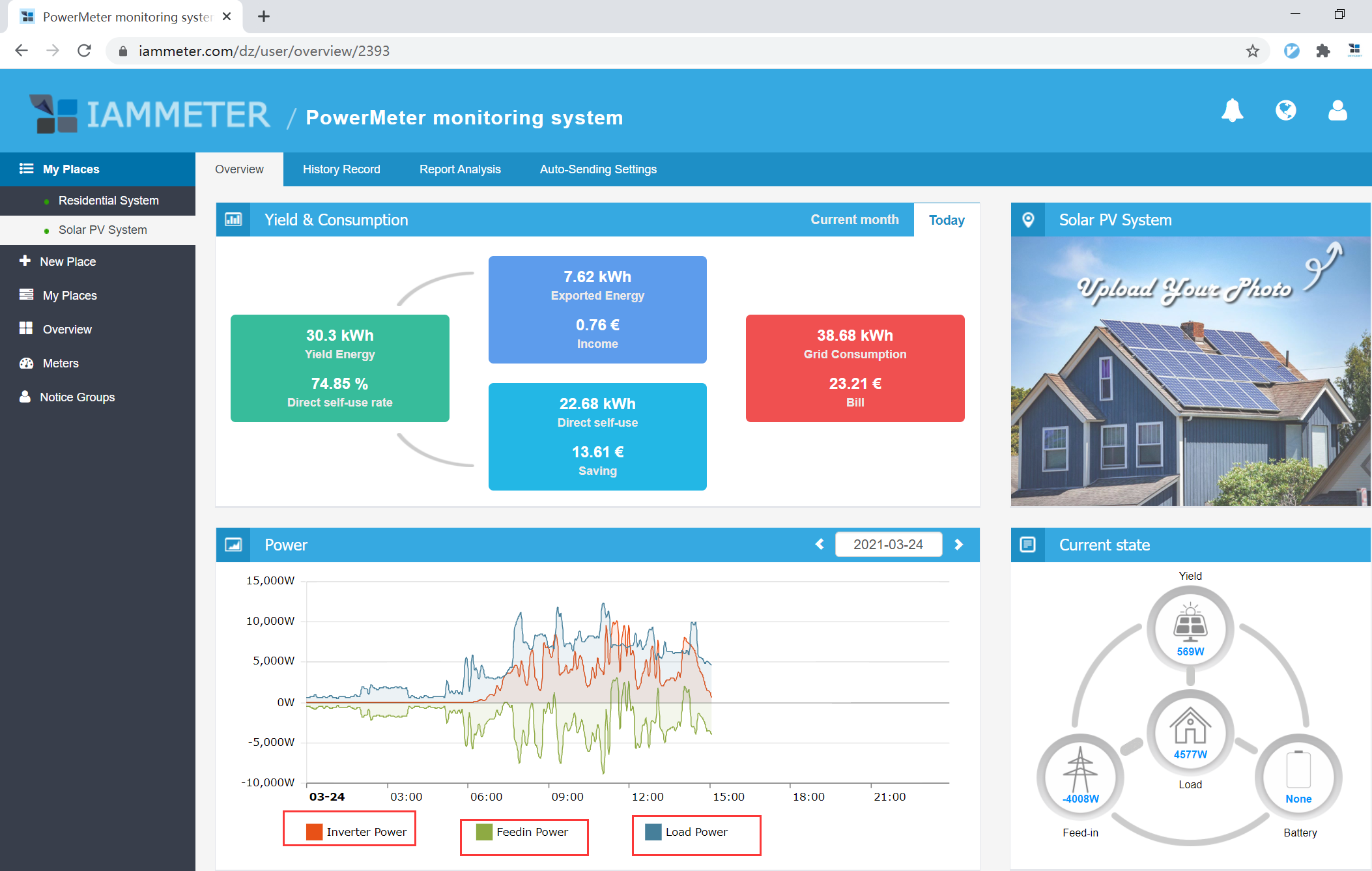Sorry, your browser does not support JavaScript!Home >

# Active Power Monitor in Solar PV System

Before you see the displays of different kinds of Active Power (Inverter Power, Feedin Power, AC storage Power, Load Power) of your solar PV system, you need to define your meter/CT type on IAMMETER.

## 1. Meter/CT Definition

According to your actual meter and CT installation, you need to define your meter/CT type on IAMMETER, as "Grid", "Inverter", "AC storage" or "Load". See below picture, and make sure that K → L direction marked in the picture is same with it marked at the bottom of CT.

1. The meter/CT type is "Grid" if you clamp this CT onto Grid side;
2. The meter/CT type is "Inverter" if you clamp this CT onto Solar Inverter side;
3. The meter/CT type is "AC Storage" if you clamp this CT onto AC Storage side;
4. The meter/CT type is "Load" if you clamp this CT onto Load side. This option is for the application that you need to monitor the power usage specific load, such as an air-conditioner. It is not to monitor the power usage of all loads because the total load power can be calculated by grid power, inverter power and AC storage power.## 2. Set your meter/CT type

See below picture, you can set your meter/CT type here.## 3. Different Active Power

In the overview webpage of solar PV system on IAMMETER, it displays three Active Power as below,

Inverter Power, Feedin Power and Load Power.

See below picture for displays of Inverter Power, Feedin Power and Load Power.### 3.1 Inverter Power

Inverter power is measured by the meter&CT on solar inverter side. It is always positive.

### 3.2 Feedin Power

Feedin power and grid power can be both measured by the meter/CT on grid side since the meter is bi-directional. Feedin power is the opposite of grid power. For instance, if grid power is 5W, the feedin power is -5W.

When feedin power＜0 (grid power＞0), it is consuming power from grid; when feedin power＞0 (grid power＜0), it is feeding power into grid.

### 3.3 AC Storage Power

AC storage power is measured by meter/CT on AC storage side. When it is positive, it is charging the battery; when it is negative, the batter is discharging.

Load power is not measured by meter/CT on load side because even if you clamp CT onto load side, the measurement is not taken into calculation. IAMMETER calculates "load power" by below formula,

Load Power= Inverter Power + Grid Power - AC Storage Power

Here is an instance for calculation of load power,

At a certain moment,

Inverter Power = 4kW, which means the power generated by solar PV system is 4kW at the moment;

FeedIn Power = 2kW (Grid Power = -2kW), which means solar PV system is exporting 2kW to the grid at the moment;

AC Storage Power = 1kW, which means solar PV system is charging 1kW to battery at the moment.

So, at the moment,

Load Power = 4kW-2kW-1kW = 1kW

Top